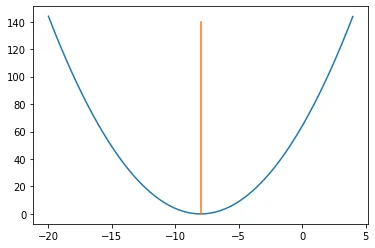## 实例展示及梯度下降算法说明

import matplotlib.pyplot as plt
import numpy as np
x = np.arange(-20, 4, 0.01)
y = (x + 8)*(x+8)
plt.plot(x,y)
plt.plot([-8,-8],[0,140])### 步骤3：让我们执行2次梯度下降迭代看看

#初始化 x0 = -3, rate = 0.01,dy/dx = 2(x+8)

#第一次迭代
# x1 = x0 - rate*(dy/dx) = -3 - 0.01*(2*(-3+8)) = -3.1
#第二次迭代
# x2 = x1 - rate*(dy/dx) = -3.1 - 0.01*(2*(-3.1+8)) = -3.198



## Python的梯度下降

### 步骤1：初始化数值

initx = -3#算法以x = -3的
rate = 0.01#学习速率
cutoff = 0.000001 #精度，这将告诉我们何时停止算法
previous_step_size = 1#
max_iters = 100000#最大迭代次数
iters = 0 #迭代计数器
df = lambda x:2 *(x + 8)#我们函数的斜率，梯度方向



### 步骤2：循环并找到最小值


while previous_step_size > cutoff and iters < max_iters:
prev_x = initx #Store current x value in prev_x
initx -= rate * df(prev_x) #Grad descent
previous_step_size = abs(initx - prev_x) #Change in x
iters = iters+1 #iteration count
print("迭代次数",iters,"\nX 值为：",initx) #Print iterations

print("获得最小值：", initx)


迭代次数 1
X 值为： -3.1

X 值为： -3.198

X 值为： -3.29404

X 值为： -3.3881592

X 值为： -3.480396016
···

X 值为： -7.883313642475223

X 值为： -7.885647369625718

X 值为： -7.8879344222332035

X 值为： -7.890175733788539

X 值为： -7.892372219112769

X 值为： -7.894524774730513

X 值为： -7.8966342792359026

X 值为： -7.898701593651184

X 值为： -7.900727561778161
····

X 值为： -7.999944830027104

X 值为： -7.999945933426562

X 值为： -7.999947014758031

X 值为： -7.99994807446287

X 值为： -7.999949112973613

X 值为： -7.99995013071414

X 值为： -7.999951128099857